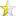Jun 21, 2013 (last update: Jun 25, 2013)

# Recursion### Recursion

What is recursion? The simple answer is, it’s when a function calls itself. But how does this happen? Why would this happen, and what are its uses?

When we talk about recursion, we are really talking about creating a loop. Let’s start by looking at a basic loop.

 ``123`` ``````for(int i=0; i<10; i++) { cout << "The number is: " << i << endl; }``````

For those who don’t yet know, this basic loop displays the sentence, "The number is: " followed by the value of ‘i’. Like this.
 ``` The number is: 0 The number is: 1 The number is: 2 The number is: 3 The number is: 4 The number is: 5 The number is: 6 The number is: 7 The number is: 8 The number is: 9 ```

Inside the ‘for loop’ declaration we have the integer variable ‘i’ and have its starting value of 0. So the first time the sentence is displayed it reads, "The number is: 0". The part of the ‘for loop’ declaration that is ‘i++’ tells the program that each time the loop repeats, the value of ‘i’ should be increased by 1. So, the next time the sentence is displayed it reads, "The number is: 1".
This cycle will continue to repeat for as long as the value of ‘i’ is less than 10. The last sentence displayed would read, "The number is: 9". As you can see the basic ‘for loop’ has three parts to its declaration, a starting value, what must remain true in order to continue repeating, and a modifying expression. Everything that is contained within the {braces} is what the program performs. Cout stands for console out, and prints words or characters to the screen.
So what does this have to do with recursion? Remember recursion is a loop. What if I did not want to just print a message to the screen? A loop can be used to perform other tasks as well.

In the following code is the same loop as above only now it is being used to call a function.

 ``123456789101112131415`` ``````#include using namespace std; void numberFunction(int i) { cout << "The number is: " << i << endl; } int main() { for(int i=0; i<10; i++) { numberFunction(i); } return 0; }``````

I have declared a void function, which means it returns nothing, and takes a parameter of ‘int i’. The function is named ‘numberFunction’ and as you can see, all it does is display the sentence, "The number is: " followed by the current value of ‘i’. The function is called into use by the ‘for loop’, which continually calls the function as long as the value of ‘i’ is less than 10.

Now with recursion, we won’t need to use a ‘for loop’ because we will set it up so that our function calls itself. Let’s recreate this same program one more time, only this time we will do it without a ‘for loop’. We will use a recursion loop instead, like this.

 ``123456789101112131415161718`` ``````#include using namespace std; void numberFunction(int i) { cout << "The number is: " << i << endl; i++; if(i<10) { numberFunction(i); } } int main() { int i = 0; numberFunction(i); return 0; }``````

We did it! We used recursion! You can see the call to ‘numberFunction’ is made only once in the main part of the program but it keeps getting called again and again from within the function itself, for as long as ‘i’ is less than 10.